DraftKings World Series Odds
+390
3.9 to 1Yankees
16.4% implied probability

+390
3.9 to 1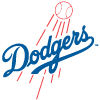Dodgers
16.4% implied probability

+1100
11 to 1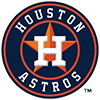Astros
6.7% implied probability

+1300
13 to 1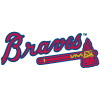Braves
5.7% implied probability

No changes have been recorded yet.
+1500
15 to 1Twins
5.0% implied probability

+1700
17 to 1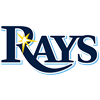Rays
4.5% implied probability

+2000
20 to 1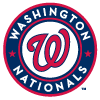Nationals
3.8% implied probability

+2000
20 to 1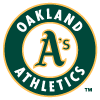Athletics
3.8% implied probability

+2000
20 to 1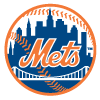Mets
3.8% implied probability

No changes have been recorded yet.
+2200
22 to 1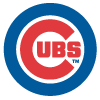Cubs
3.5% implied probability

+2200
22 to 1Indians
3.5% implied probability

+2500
25 to 1Phillies
3.1% implied probability

+2500
25 to 1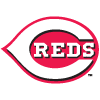Reds
3.1% implied probability

+3500
35 to 1Brewers
2.2% implied probability

+3500
35 to 1Angels
2.2% implied probability

+3500
35 to 1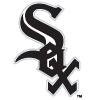White Sox
2.2% implied probability

+4000
40 to 1Rockies
2.0% implied probability

+4000
40 to 1Red Sox
2.0% implied probability

No changes have been recorded yet.
+4000
40 to 1Padres
2.0% implied probability

+4000
40 to 1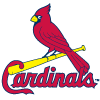Cardinals
2.0% implied probability

+5000
50 to 1Diamondbacks
1.6% implied probability

No changes have been recorded yet.
+7000
70 to 1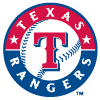Rangers
1.1% implied probability

+8000
80 to 1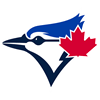Blue Jays
1.0% implied probability

+15000
150 to 1Pirates
0.5% implied probability

+15000
150 to 1Giants
0.5% implied probability

No changes have been recorded yet.
+24000
240 to 1Marlins
0.3% implied probability

+30000
300 to 1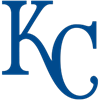Royals
0.3% implied probability

+30000
300 to 1Tigers
0.3% implied probability

+30000
300 to 1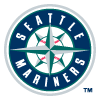Mariners
0.3% implied probability

+30000
300 to 1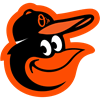Orioles
0.3% implied probability